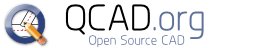QCAD Bugtracker

• Status Assigned
• Percent Complete
0%
• Task Type Feature Request
• Category QCAD (main)
• Assigned To
• Operating System All
• Severity Low
• Priority Very Low
• Reported Version 3.16.5
• Due in Version Undecided
• Due Date Undecided
• CVH (16.04.2018)
• Private
Attached to Project: QCAD Bugtracker
Opened by Baumi - 08.03.2017

FS#1555 - Export list of points.

Hello
It would be great when you can add a function to export a selected object as a list of points.
Is it possible to add?

Autodes DWG TrueView 2017 has this function and it is very useful.
Here you can see a screenshot of this function.

Andrew commented on 09.03.2017 07:44

Thanks for your feature request.
To increase the chances of this being implemented, please provide sample input / output and explain why such a feature might be useful to you and others. Thanks.

Baumi commented on 10.03.2017 06:59

We use the exported List of points as input for a Kuka Robot System. We receive CAD drawings, select some regions of it and create trajectories for the robot.

Baumi commented on 10.03.2017 07:01

output could look like this:

at point  X=  82.1944  Y= 424.7488  Z=   0.0000
at point  X=  82.1944  Y= 423.2455  Z=   0.0000
at point  X=  82.1944  Y= 420.6953  Z=   0.0000
at point  X=  82.1944  Y= 419.3444  Z=   0.0000
at point  X=  82.1944  Y= 415.3822  Z=   0.0000
at point  X=  82.1944  Y= 413.3255  Z=   0.0000
at point  X=  82.1944  Y= 413.1276  Z=   0.0000
at point  X=  82.2079  Y= 413.0771  Z=   0.0000
at point  X=  82.2258  Y= 413.0100  Z=   0.0000
at point  X=  82.2357  Y= 412.9729  Z=   0.0000
at point  X=  82.2477  Y= 412.9280  Z=   0.0000
at point  X=  82.2557  Y= 412.8981  Z=   0.0000
at point  X=  82.3728  Y= 412.4594  Z=   0.0000
at point  X=  87.4300  Y= 393.5195  Z=   0.0000
at point  X=  88.3974  Y= 389.8966  Z=   0.0000
at point  X=  88.6478  Y= 388.9589  Z=   0.0000
at point  X=  88.7407  Y= 388.6106  Z=   0.0000
at point  X=  88.8186  Y= 388.2955  Z=   0.0000
at point  X=  88.8646  Y= 388.0857  Z=   0.0000
at point  X=  88.9089  Y= 387.8604  Z=   0.0000
at point  X=  88.9427  Y= 387.6668  Z=   0.0000
at point  X=  88.9700  Y= 387.4917  Z=   0.0000
at point  X=  88.9972  Y= 387.2924  Z=   0.0000
at point  X=  89.0151  Y= 387.1421  Z=   0.0000
Andrew commented on 10.03.2017 07:19

Thanks for the details.

What coordinates would get exported for a line? Start point and end point? All points on the line with a given interval? How about arcs, circles, splines, etc?
How can you later determine which coordinates were part of the same line? Or is that irrelevant for your use case? Thanks.

CVH commented on 16.04.2018 11:02

I was looking for a similar feature.
Plotting Baumi (Baumfaust) list there is none arbitrary reference to what point is listed.
Baumi (Baumfaust) could consider G-code as an option.

It would serve me:
As above with fixed or percentile spacing as a textlist.
With multi lines or closed lines the fixed or percentile spacing can be per segment or per total lenght.
Fixed spacing is even possible dx appart orthogonal along a defined ray. Serves for integration.

OR/AND

As kinds & nodes as a textlist:
Lines:
-Line
-Startpoint XY(Z)
-Endpoint XY(Z)

Arcs:
-Arc
-Specific data of the arc (radius,center, startangle, endangle)
OR
-Polyline (lines) from 'exploding' eg interpolation with certain approximation

Polylines of line pieces:
-Polyline
-Startpoint XY(Z) (node0)
-Node1 XY(Z)
-...
-NodeN-1 XY(Z)
-Endpoint XY(Z) (NodeN)

Polylines of line & arc pieces or merely arc pieces:
-Polyline
-Any startpoint of an arc is followed by a bulgefactor (linesegments have no bulge)
OR
-followed by specific data of the arc (radius,center, startangle, endangle)
OR
-Polyline (lines)
-Line segments from 'exploding' eg interpolation with certain approximation

Circle
-Circle
-Specific data of the circle (radius,center)
OR
-Polyline (arcs)
-Specific data of

1. 4 arcs (radius,center, startangle, endangle)

OR

1. N arcs (radius,center, startangle, endangle)

OR
-Polyline (lines)
-Line segments from 'exploding' eg interpolation with certain approximation

Ellipse:
-Ellipse
-Specific data of the ellipse
OR
-Polyline (lines or/and/mixed arcs) from exploding with certain approximation
With arcs or mixed with arcs the tangentially nature of the segments is preserved

Splines
-Spline control//fit
-Specific data of the spline
OR
-Polyline (lines or/and/mixed arcs) from exploding with certain approximation
With arcs or mixed with arcs the tangentially nature of the segments is preserved

Same rules can be applied for further sorts of entities.

In tandem with importing such a list it would be of great benifit.

CVH

CVH commented on 14.05.2018 15:33

Baumi,

If you are still listening.
If you turn your object into a polyline.
You can use the script Misc\Script Examples\'Import/Export'\Dump Polyline.
The dump will be in the Message box.
If you want arc info you could change the script yourself to output the bulge too.
...scripts\Misc\Examples\IOExamples\ExDumpPolyline.js

for (var k=0; k<n; k++) {
var v = entity.getVertexAt(k);
var w = entity.getBulgeAt(k);
EAction.handleUserMessage("%1,%2,%3,".arg(v.x).arg(-v.y).arg(w));
}

The math for the arc you can find here
http://www.lee-mac.com/bulgeconversion.html

Regards

Available keyboard shortcuts

• SHIFT+ALT+l Login Dialog / Logout
• SHIFT+ALT+m My searches
• SHIFT+ALT+t focus taskid search

• o open selected task
• j move cursor down
• k move cursor up